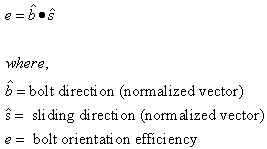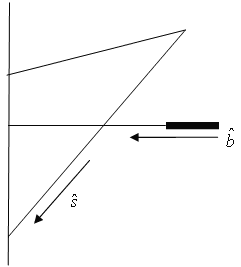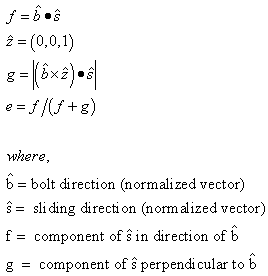The Rocscience International Conference 2021 Proceedings are now available. Read Now

Bolt Orientation Efficiency

Bolts which intersect a wedge can deform in a number of different ways, as shown in Figure 1. As a result, the mechanism of failure of a bolt can be quite complicated. In order to simplify the behavior for the purpose of including support in a limit-equilibrium wedge analysis program such as UnWedge, an empirical reinforcement efficiency factor can be used.Fig:1 Possible bolt deformation modes [Windsor, 1996]

The theoretical tensile capacity of a bolt at any point along its length, is determined from the Bolt Force Diagram, and the point at which a wedge plane intersects the bolt. This assumes that a bolt is in pure tension. However, pure tension will only occur if the bolt orientation is aligned exactly with the sliding direction or direction of movement of the wedge (see Figure 1 - case B).

If a bolt is not in pure tension, but also shear, the effective tensile capacity of the bolt will be reduced. The amount of reduction is given by the Bolt Efficiency factor, which may range between zero and one.

• If the bolt efficiency is zero, the bolt has no effect and zero force is placed into the equilibrium equations of the wedge.
• Conversely, if the efficiency is one, the bolt is in pure tension and the entire capacity is added as a force in the direction of the bolt in the equilibrium equations of the wedge.

NOTE: The Bolt Orientation Efficiency factor, if used, is ONLY applied to the Tensile Capacity of a bolt as determined from the Bolt Force Diagram. It is NOT applied to the Shear Capacity of a bolt, if the Shear Strength option is being used. For an overall summary of the bolt support force implementation in UnWedge, see the Bolt Support Force topic.

The Bolt Orientation Efficiency factor is calculated by relating the direction of sliding or movement of the wedge to the orientation of the bolt. In UnWedge 3.0 there are three different calculation methods.

Cosine Tension / Shear

This is the default method in UnWedge. With reference to Figure 2, the efficiency is calculated as:This applies to bolt deformation modes A, B, C, D (see Figure 1). For bolt deformation modes E (pure shear) or F (compression and shear), the bolt efficiency = 0.Fig.2: Bolt orientation and sliding direction used for Bolt Efficiency calculation

Linear Tension / Shear

With the Linear Tension / Shear method, the efficiency is calculated as:This applies to bolt deformation modes A, B, C, D (see Figure 1). For bolt deformation modes E (pure shear) or F (compression and shear), the bolt efficiency = 0.

UnWedge 2.0

UnWedge 2.0 was the original DOS version of UnWedge. In that version, a simplified bolt efficiency was used, in which the efficiency was either 0 or 1, depending on the deformation mode of the bolt. With reference to Figure 1:Bolt Efficiency Off

There is also the possibility that the Use Bolt Orientation Efficiency option can be turned off. If you do not wish to use Bolt Efficiency, then clear the Use Bolt Orientation Efficiency checkbox in the Bolt Properties dialog.

If Use Bolt Orientation Efficiency is OFF, then the bolt efficiency will equal 1, regardless of the bolt deformation mode (A,B,C,D,E,F -- see Figure 1).

Bolt Efficiency and Shear Strength

Finally, note that the Use Bolt Orientation Efficiency is NOT used in conjunction with the Use Shear Strength option. If you have selected the Use Shear Strength option, and the bolt deformation mode is D, E or F, so that the Bolt Shear Strength is applied, the Bolt Efficiency DOES NOT multiply the shear strength. The Bolt Efficiency is only used as a factor for the tensile bolt capacity determined from the Bolt Force Diagram.